# Discrete Control with QUBE-Servo 2

Michel Levis

Senior Application Engineer | Quanser Inc.

Today, most control systems are being implemented on digital computers, using either a PC/laptop or a microprocessor unit (MCU) like Raspberry Pi or Arduino. Gone are the days of implementing your PID on an analog circuit. However, controllers implemented on a digital computer are continuous, running on digital equipment,  and are being sampled or “discretized”. They are not, strictly speaking, true analog controllers.

In this post from my series focused on an extensive curriculum we developed for our QUBE-Servo 2 system, I will show you how we introduce the discrete control concepts to students.

#### Why perform discrete control?

When running at fast, deterministic sampling rates, the effects of implementing a continuous controller on a digital computer are negligible. On the other hand, the effects of implementing such controllers on a non-deterministic platform (i.e., non-real-time) or at lower sampling rates can be quite pronounced. In these cases, using a discrete version of the controller can yield much better performance. Discrete control analysis and design can, therefore, lead to a deeper understanding of how sampling affects system stability and how you can improve the performance of your system.

#### Overview of QUBE-Servo 2 Discrete Control Labs

The discrete control labs included in the QUBE-Servo 2 curriculum are summarized in the table below. All these labs are new, added in summer 2020. If you are working with an older version of course materials, get in touch with us and we will share the latest version with you.

 Lab Description Introduction to Discrete Control Learn how to model a digital computer, i.e., sampling, and how that affects the response. Discrete Stability Learn how to assess the stability of a system in the discrete z-domain and how sampling affects stability. Discrete Control Design Design a controller directly in the discrete domain, i.e., not a continuous controller that is discretized through sampling.

#### Introduction to Discrete Control

The main difference between a true continuous controller and one implemented on a digital computer is the sampling. The PC/laptop/MCU is connected to a data acquisition (DAQ) device that receives measured sensor signals through the analog-to-digital (A/D) converter and outputs signals through the digital-to-analog (D/A) converter at fixed sampling intervals. The interface between the DAQ and the plant when running a position controller on the QUBE-Servo 2 is shown below.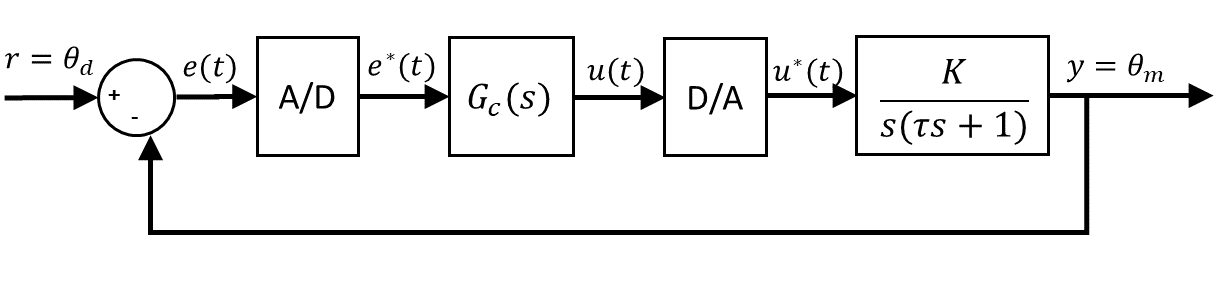The sampling can be modelled using an ideal sampler and a zero-order hold (ZOH) function, shown in the following block diagram.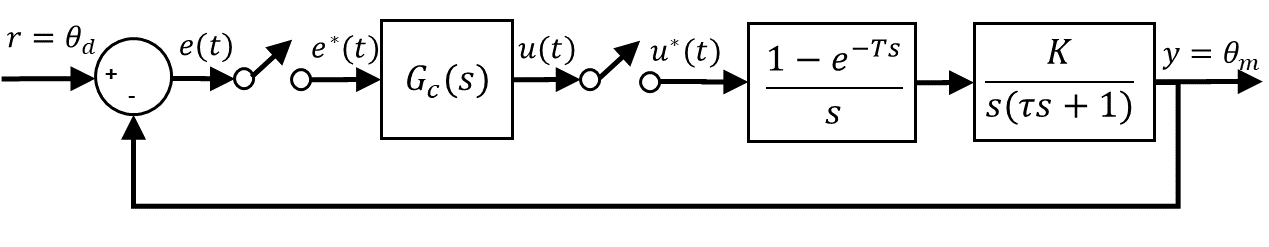This is used to investigate the effects of applying a continuous controller in a digital environment when sampling at different rates. In the lab, the QUBE-Servo 2 position control loop is run at 500 Hz and at 33.3 Hz. The degradation in the performance of the position control at the lower sampling rate is substantial and demonstrates the effect that sampling has.

The performance at this rate would be greatly improved if the controller was designed in the discrete domain. This is the main benefit of discrete control design.

#### Discrete Stability

In the previous blog post, I covered the various methods that can be used to analyze stability in the continuous domain. One of the most used methods is looking at the pole locations in the s-plane. For discrete systems, a similar method is used but in this case, the pole locations in the z-domain are assessed based on their location relative to the unit circle, as shown below.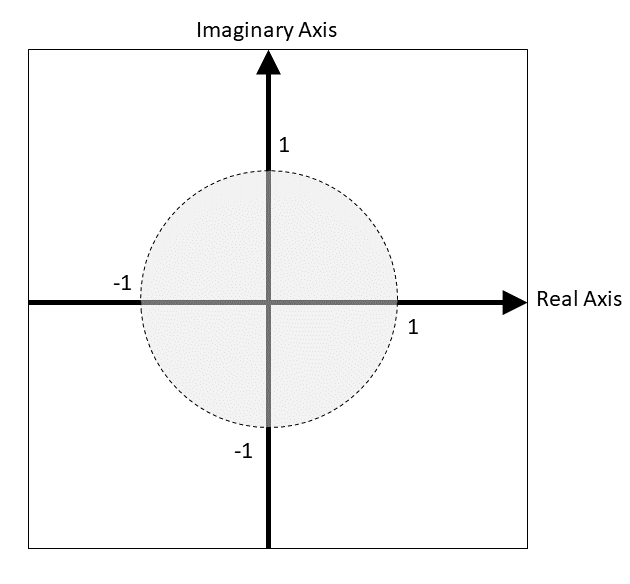In the first part of the lab, the stability of the open-loop QUBE-Servo 2 DC motor is assessed at two different sampling rates – 33.3 Hz and 500 Hz. That demonstrates how lower and higher sampling rates affect the stability of a system.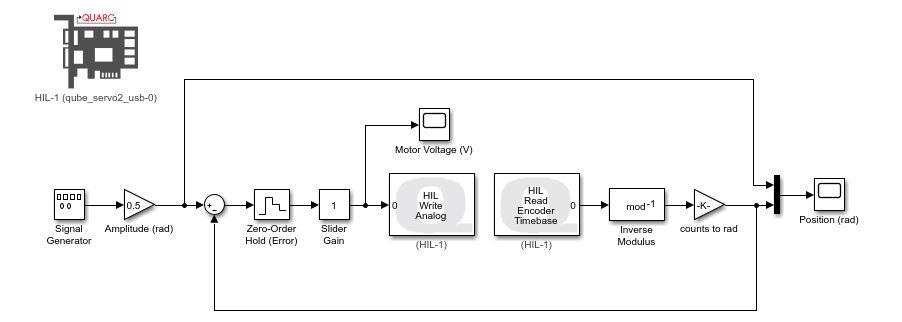In the second part of the lab, the QUBE-Servo 2 is placed in a closed-loop proportional control. The stability of the system is first examined using the Matlab Control System Designer tool for a varying range of control gain values when running at 33.3 Hz. How the gain selection affects the actual performance of the system is then tested on the servo hardware using the Quanser QUARC control software.

The advantage of the discrete stability analysis is that it takes the sampling rate into account. While the effect of the sampling can be included in the continuous stability analysis (as is done in the Robustness lab), it is often neglected.

#### Direct Discrete Design

In this lab, a discrete lead compensator is designed to control the position of the motor QUBE-Servo 2 motor at a lower sampling rate. In the previous labs, the continuous controllers were implemented digitally, using approximation techniques, i.e., sampling. In this case, the controller is designed directly in the z-domain for a specific sampling interval. The control output is based on the current sampling instance and is not prone to approximation errors.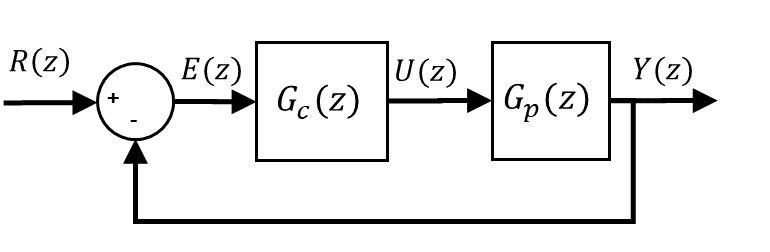The lead compensator is designed using the Matlab Control System Designer tool to meet a set of time-domain specifications (e.g. settling time, percentage overshoot) for a sampling rate of 33.3 Hz. Once the simulation results match the desired requirements, the lead control is implemented on the actual QUBE-Servo 2 using the Quanser QUARC software. The sample result below shows the position control response when running at this rate (default results – not designed for specifications).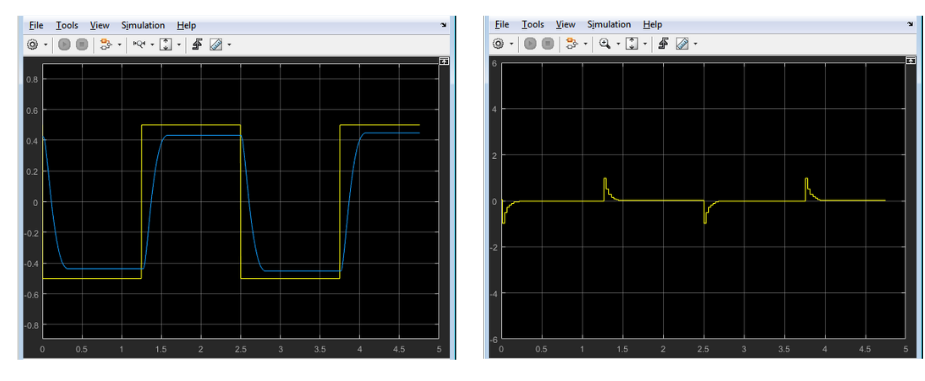This demonstrates the advantage of a direct discrete control design – you can get better performance despite running at lower sampling rates or on non-deterministic systems. The disadvantage is that you have to redesign your controller every time the sampling rate is changed.

#### Final Notes

Discrete control design can lead to better performance, but continuous controllers running on a digital computer at high, deterministic sampling rates typically do not experience issues with the performance due to sampling approximations. But if you are designing control for a high-end application or one that is ran on an MCU with non-deterministic (i.e., not real-time) performance, then these techniques have some benefit.